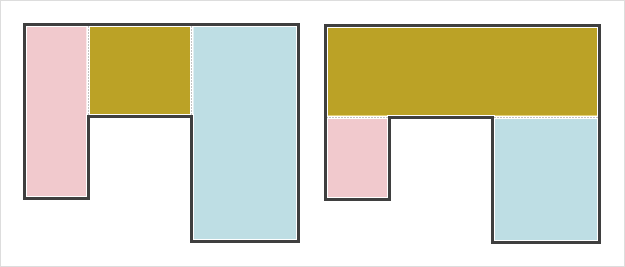# Square Footage Calculator

 Rectangle 1 Length ft. in. Width ft. in. Rectangle 2 (Optional) Length ft. in. Width ft. in. Rectangle 3 (Optional) Length ft. in. Width ft. in. sq. ft.

If you measure in feet and inches, you have to handle two units of measurement when calculating area. Since inches are 1/12 of a foot, they do not lend themselves to easy decimal conversions as do centimeters and meters. E.g., 1 meter and 60 centimeters can be written equivalently as 1.6 meters, but 1 foot and 6 inches does not equate to 1.6 feet. Since 6 inches is half a foot, 1'6" is actually equal to 1.5 feet.

There are two approaches to hand calculate the area of a rectangle when the dimensions are given in feet and inches. For convenience, you can also use the calculator on the left.

#### Method 1: Convert to Feet

First convert the inches to their decimal equivalents in feet using the chart below.

 1 in. = 0.08333 ft. 7 in. = 0.58333 ft. 2 in. = 0.16667 ft. 8 in. = 0.66667 ft. 1 in. = 0.25 ft. 9 in. = 0.75 ft. 4 in. = 0.33333 ft. 10 in. = 0.83333 ft. 5 in. = 0.41667 ft. 11 in. = 0.91667 ft. 6 in. = 0.5 ft.

Once you have converted the inches to feet, add it to the whole number foot measurement to get the total feet. Then multiply the width and length of the rectangle to get the total area in square feet.

Example: A room is 16 feet 9 inches long and 12 feet 5 inches wide. In pure feet, this is equivalent to 16.75 feet long by 12.41667 feet wide. So the total area is (16.75)(12.41667) = 207.9792 square feet, or about 208 square feet.

#### Method 2: Convert to Inches First, Convert to Feet Last

Convert the dimensions of the room to inches using the rule that 1 foot = 12 inches. Multiply the width and length to obtain the area of the room to square inches, then divide by 144 to convert it to square feet.

Example: A room is 16 feet 9 inches long and 12 feet 5 inches wide. In inches, the length and width are

12(16) + 9 = 201 inches long
12(12) + 5 = 149 inches wide.

Since (201)(149) = 29949, the area of the room is 29949 square inches. And since 29949/144 = 207.9792, the area of the room is around 208 square feet.

#### Tips for Oddly Shaped Rooms

If a room is not rectangular, you can find its area by dividing the shape into smaller rectangles, calculating their areas, then adding them to find the total area. There is often more than one way to partition a room into rectangles; you will arrive at the same final answer no matter how you divide up the area.